Next: Gibbs Free Energy Up: Classical Thermodynamics Previous: Enthalpy

# Helmholtz Free Energy

Suppose thatandare the are the two independent parameters that specify the system. Because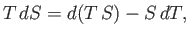(6.100)

we can rewrite Equation (6.82) in the form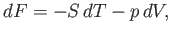(6.101)

where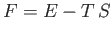(6.102)

is termed the Helmholtz free energy.

Proceeding as before, we write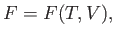(6.103)

which implies that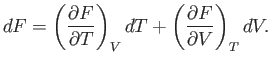(6.104)

Comparison of Equations (6.101) and (6.104) yields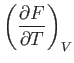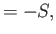(6.105)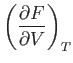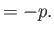(6.106)

We also know that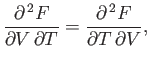(6.107)

or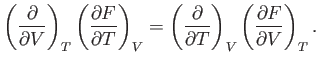(6.108)

Thus, it follows from Equations (6.105) and (6.106) that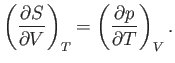(6.109)Next: Gibbs Free Energy Up: Classical Thermodynamics Previous: Enthalpy
Richard Fitzpatrick 2016-01-25